# Argument An argument is a sequence of statements

• Slides: 34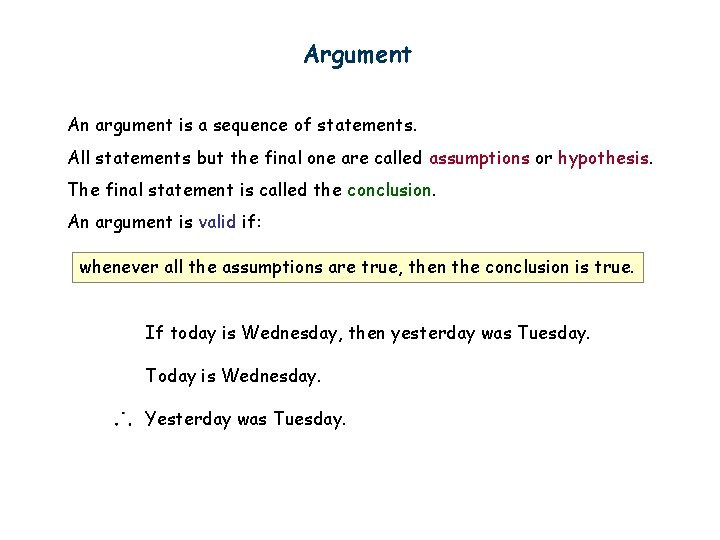Argument An argument is a sequence of statements. All statements but the final one are called assumptions or hypothesis. The final statement is called the conclusion. An argument is valid if: whenever all the assumptions are true, then the conclusion is true. If today is Wednesday, then yesterday was Tuesday. Today is Wednesday. Yesterday was Tuesday.Modus Ponens If p then q. p q If bandh, then class cancelled. Bandh. Class cancelled. assumptions p T T F F q T F p→q T F T T p T T F F conclusion q T F Modus ponens is Latin for “method of affirming”.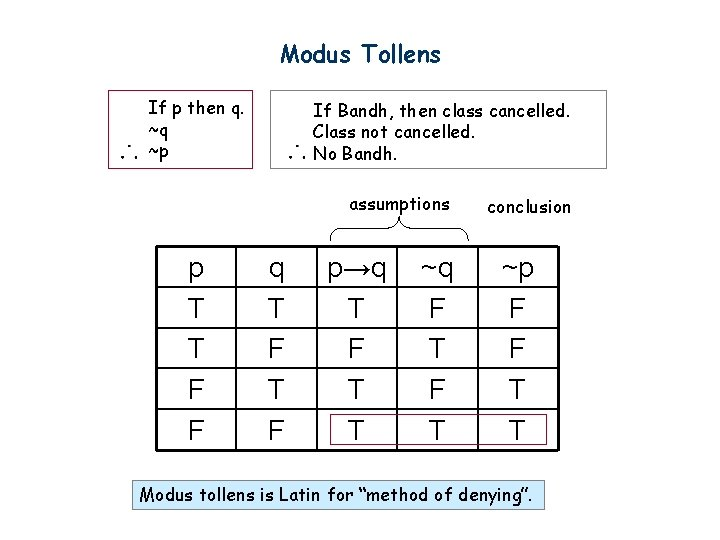Modus Tollens If p then q. ~q ~p If Bandh, then class cancelled. Class not cancelled. No Bandh. assumptions p T T F F q T F p→q T F T T ~q F T conclusion ~p F F T T Modus tollens is Latin for “method of denying”.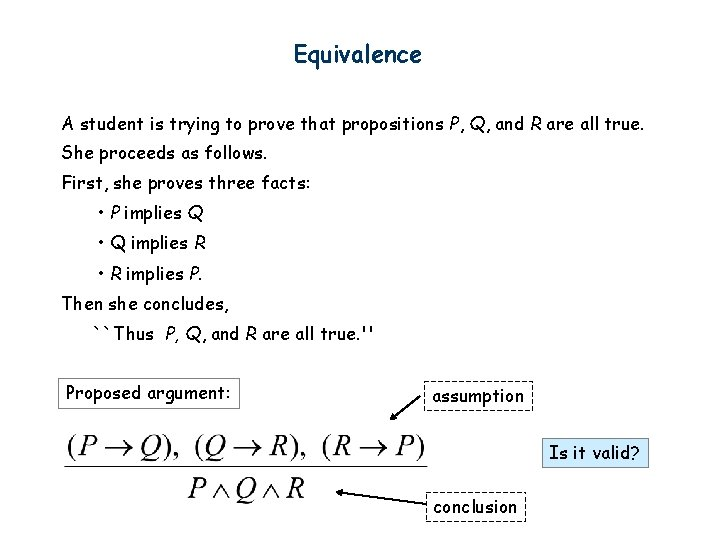Equivalence A student is trying to prove that propositions P, Q, and R are all true. She proceeds as follows. First, she proves three facts: • P implies Q • Q implies R • R implies P. Then she concludes, ``Thus P, Q, and R are all true. '' Proposed argument: assumption Is it valid? conclusion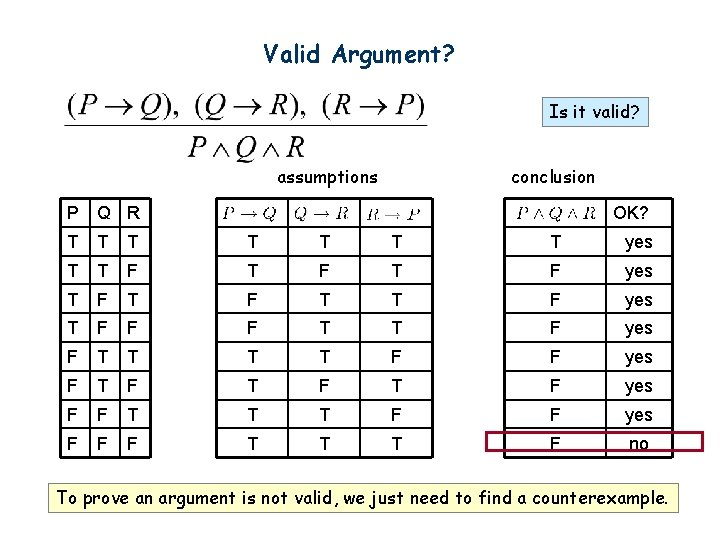Valid Argument? Is it valid? assumptions conclusion P Q R OK? T T T T yes T T F T F yes T F T T F yes T F F F T T F yes F T T F F yes F T F T F yes F F T T T F F yes F F F T T T F no To prove an argument is not valid, we just need to find a counterexample.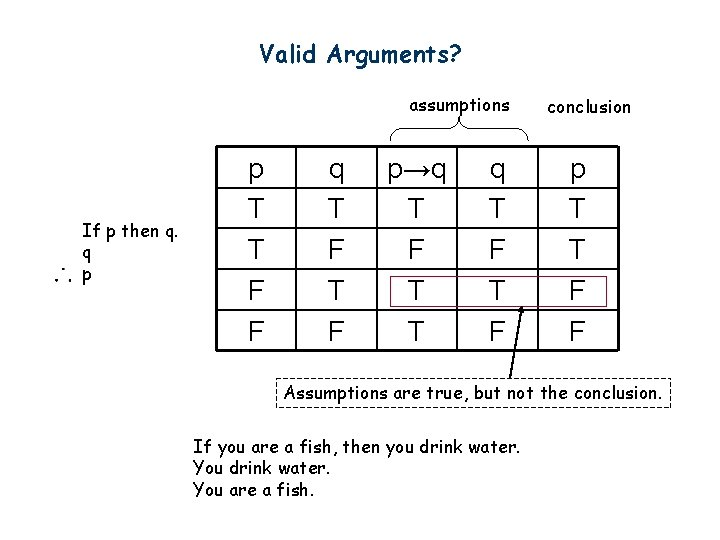Valid Arguments? assumptions If p then q. q p p T T F F q T F p→q T F T T q T F conclusion p T T F F Assumptions are true, but not the conclusion. If you are a fish, then you drink water. You are a fish.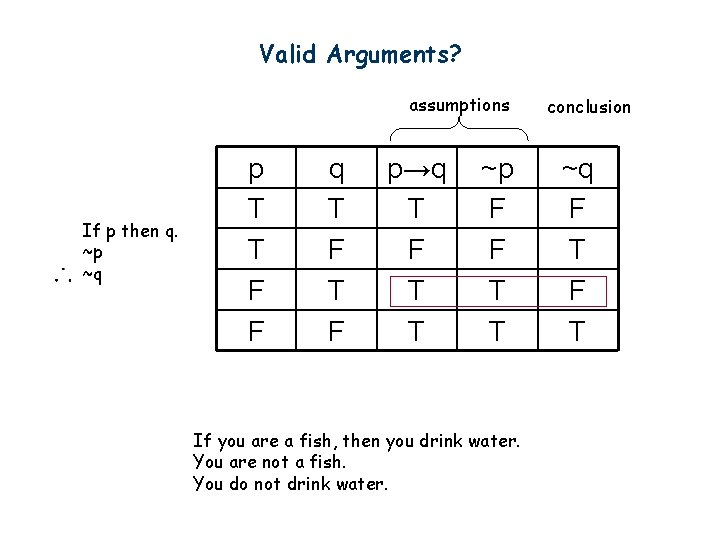Valid Arguments? assumptions If p then q. ~p ~q p T T F F q T F p→q T F T T ~p F F T T If you are a fish, then you drink water. You are not a fish. You do not drink water. conclusion ~q F T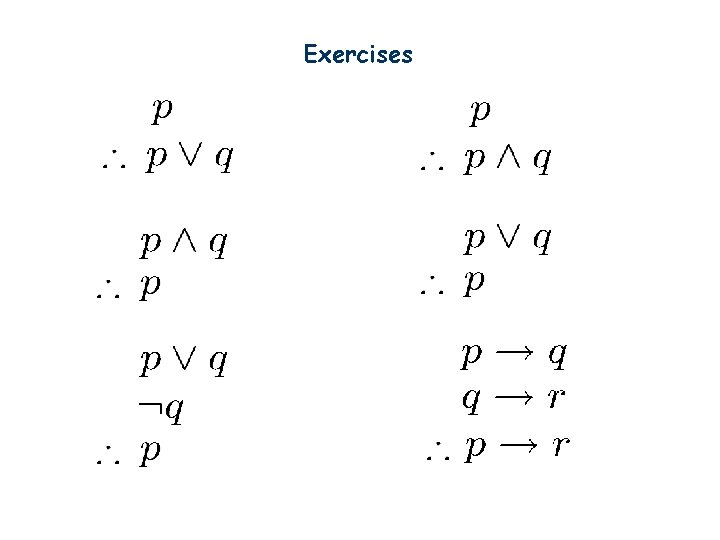ExercisesMore Exercises Valid argument True conclusion Valid argument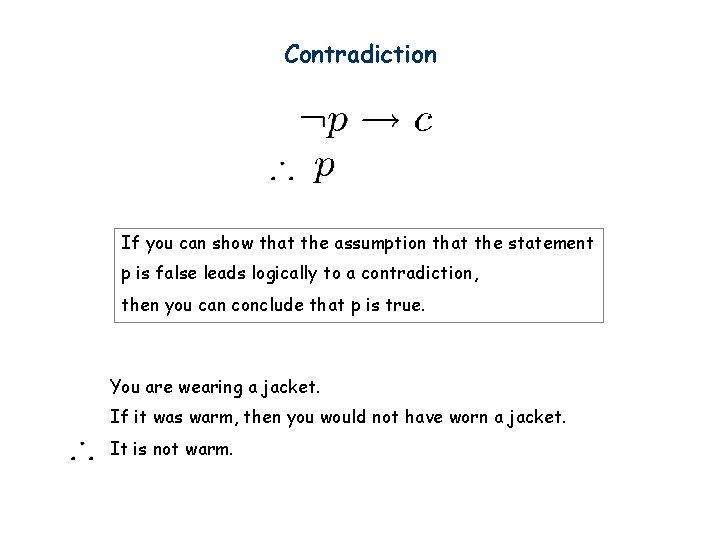Contradiction If you can show that the assumption that the statement p is false leads logically to a contradiction, then you can conclude that p is true. You are wearing a jacket. If it was warm, then you would not have worn a jacket. It is not warm.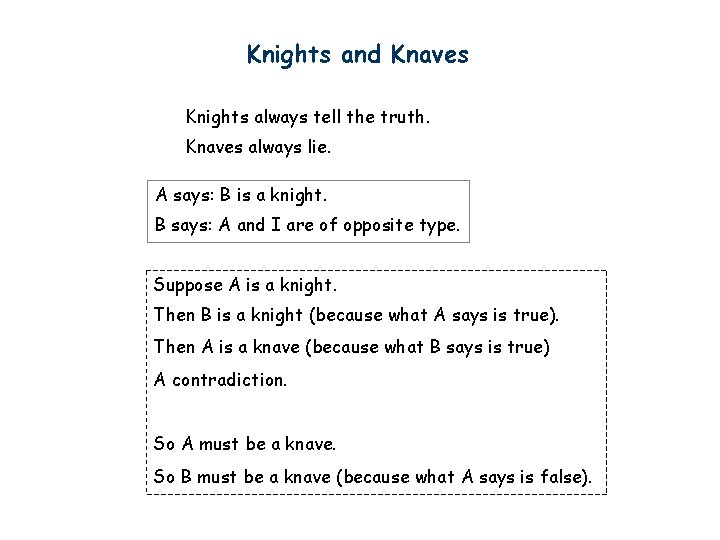Knights and Knaves Knights always tell the truth. Knaves always lie. A says: B is a knight. B says: A and I are of opposite type. Suppose A is a knight. Then B is a knight (because what A says is true). Then A is a knave (because what B says is true) A contradiction. So A must be a knave. So B must be a knave (because what A says is false).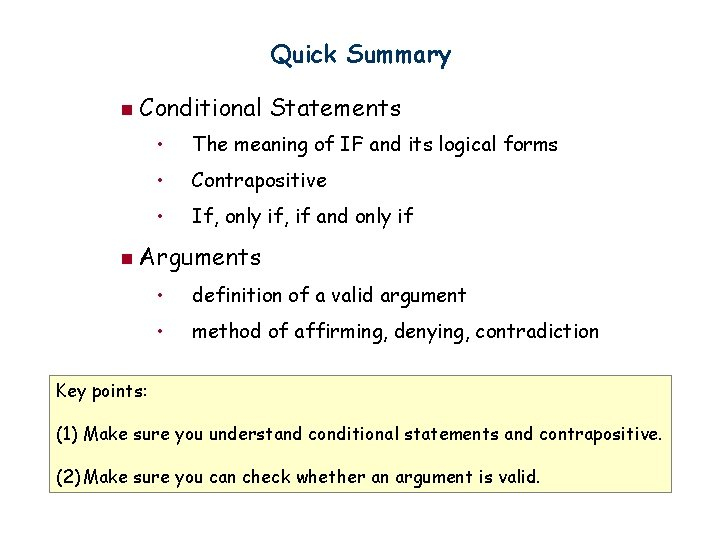Quick Summary n Conditional Statements • The meaning of IF and its logical forms • Contrapositive • If, only if, if and only if n Arguments • definition of a valid argument • method of affirming, denying, contradiction Key points: (1) Make sure you understand conditional statements and contrapositive. (2) Make sure you can check whether an argument is valid.Which is true? Which is false? “The sentence below is false. ” “The sentence above is true. ”Limitation of Propositional Logic Propositional logic – logic of simple statements How to formulate Pythagoreans’ theorem using propositional logic? c b a How to formulate the statement that there are infinitely many primes?Predicates are propositions (i. e. statements) with variables Example: P (x, y) : : = x + 2 = y x = 1 and y = 3: P(1, 3) is true x = 1 and y = 4: P(1, 4) is false P(1, 4) is true When there is a variable, we need to specify what to put in the variables. The domain of a variable is the set of all values that may be substituted in place of the variable.Set To specify the domain, we often need the concept of a set. Roughly speaking, a set is just a collection of objects. Some examples R Set of all real numbers Z Set of all integers Q Set of all rational numbers Given a set, the (only) important question is whether an element belongs to it. means that x is an element of A (pronounce: x in A) means that x is not an element of A (pronounce: x not in A) Sets can be defined explicitly: e. g. {1, 2, 4, 8, 16, 32, …}, {CSC 1130, CSC 2110, …}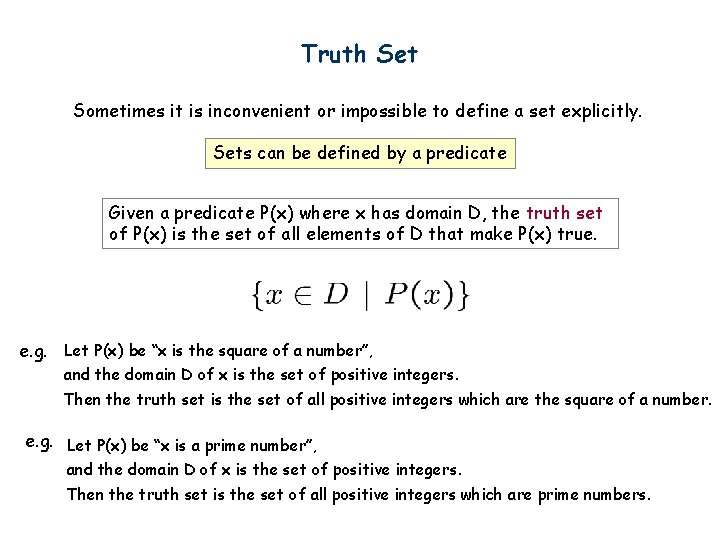Truth Set Sometimes it is inconvenient or impossible to define a set explicitly. Sets can be defined by a predicate Given a predicate P(x) where x has domain D, the truth set of P(x) is the set of all elements of D that make P(x) true. e. g. Let P(x) be “x is the square of a number”, and the domain D of x is the set of positive integers. Then the truth set is the set of all positive integers which are the square of a number. e. g. Let P(x) be “x is a prime number”, and the domain D of x is the set of positive integers. Then the truth set is the set of all positive integers which are prime numbers.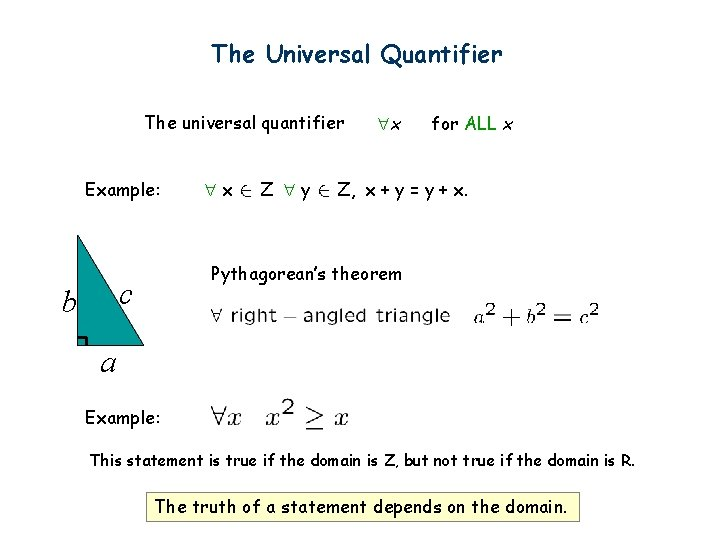The Universal Quantifier The universal quantifier Example: Z y for ALL x Z, x + y = y + x. Pythagorean’s theorem c b x x a Example: This statement is true if the domain is Z, but not true if the domain is R. The truth of a statement depends on the domain.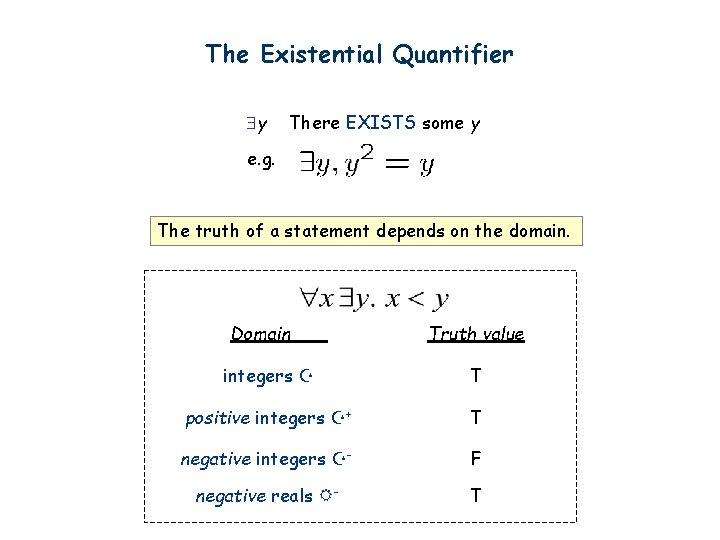The Existential Quantifier y There EXISTS some y e. g. The truth of a statement depends on the domain. Domain Truth value integers T positive integers + T negative integers - F negative reals - TTranslating Mathematical Theorem Fermat (1637): If an integer n is greater than 2, then the equation an + bn = cn has no solutions in non-zero integers a, b, and c. Andrew Wiles (1994) http: //en. wikipedia. org/wiki/Fermat's_last_theorem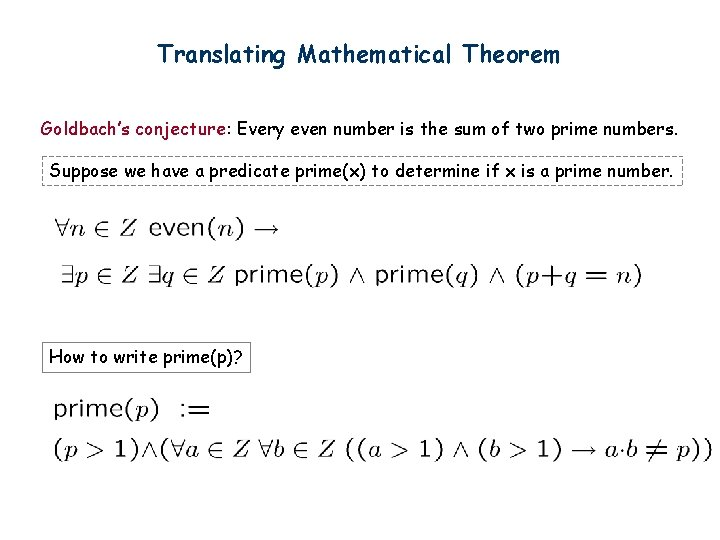Translating Mathematical Theorem Goldbach’s conjecture: Every even number is the sum of two prime numbers. Suppose we have a predicate prime(x) to determine if x is a prime number. How to write prime(p)?l Quantifiers l Negation l Multiple quantifiers l Arguments of quantified statements l (Optional) Important theorems, applicationsNegations of Quantified Statements Everyone likes football. What is the negation of this statement? Not everyone likes football = There exists someone who doesn’t like football. (generalized) De. Morgan’s Law Say the domain has only three values. The same idea can be used to prove it for any number of variables, by mathematical induction.Negations of Quantified Statements There is a plant that can fly. What is the negation of this statement? Not exists a plant that can fly = every plant cannot fly. (generalized) De. Morgan’s Law Say the domain has only three values. The same idea can be used to prove it for any number of variables, by mathematical induction.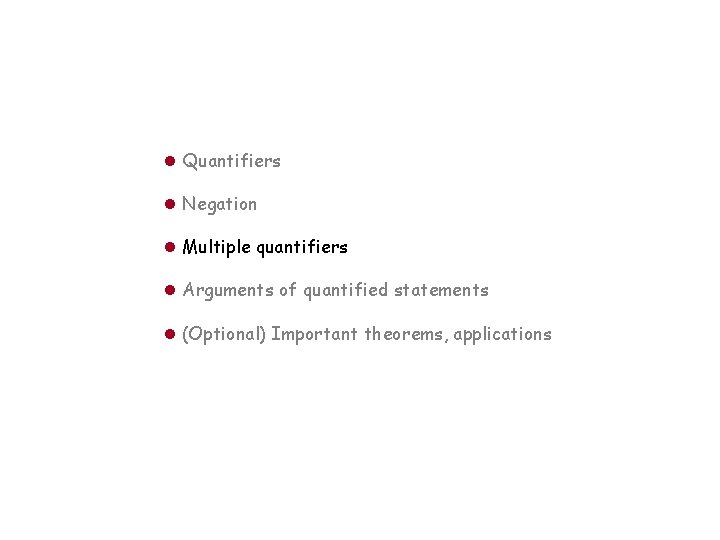l Quantifiers l Negation l Multiple quantifiers l Arguments of quantified statements l (Optional) Important theorems, applicationsOrder of Quantifiers There is an anti-virus program killing every computer virus. How to interpret this sentence? For every computer virus, there is an anti-virus program that kills it. • For every attack, I have a defense: • against MYDOOM, use Defender • against ILOVEYOU, use Norton • against BABLAS, use Zonealarm … is expensive!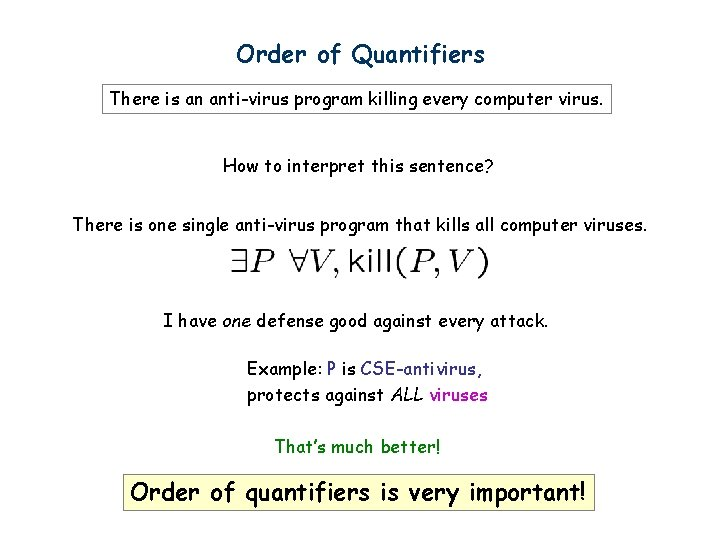Order of Quantifiers There is an anti-virus program killing every computer virus. How to interpret this sentence? There is one single anti-virus program that kills all computer viruses. I have one defense good against every attack. Example: P is CSE-antivirus, protects against ALL viruses That’s much better! Order of quantifiers is very important!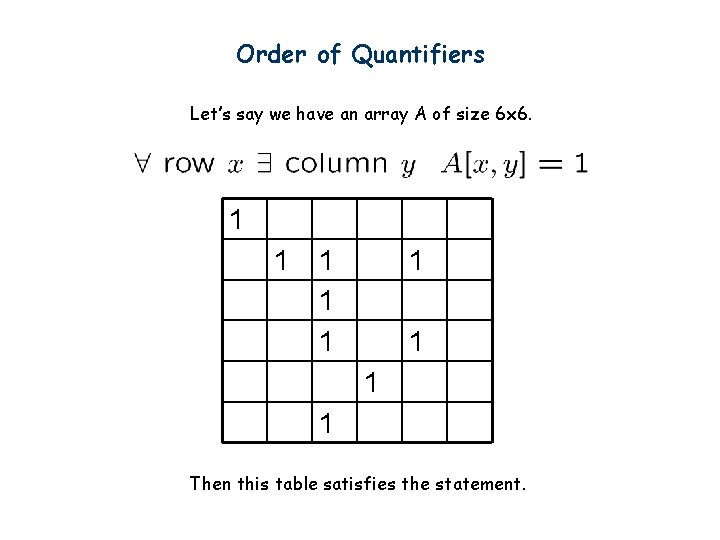Order of Quantifiers Let’s say we have an array A of size 6 x 6. 1 1 1 1 1 Then this table satisfies the statement.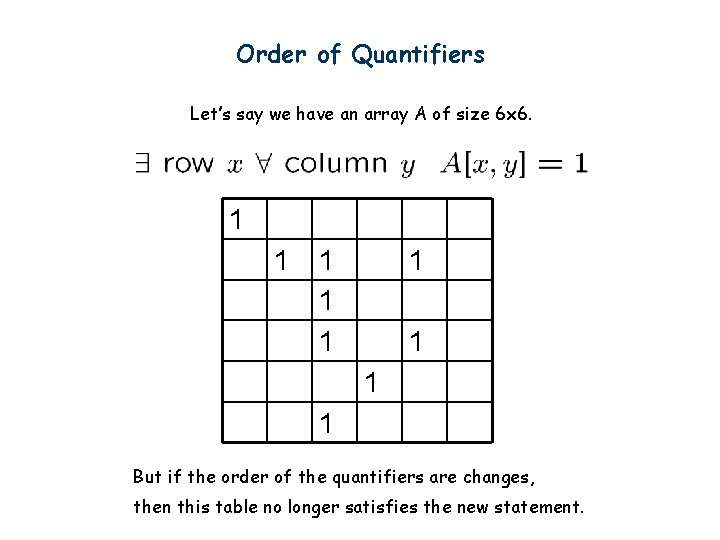Order of Quantifiers Let’s say we have an array A of size 6 x 6. 1 1 1 1 1 But if the order of the quantifiers are changes, then this table no longer satisfies the new statement.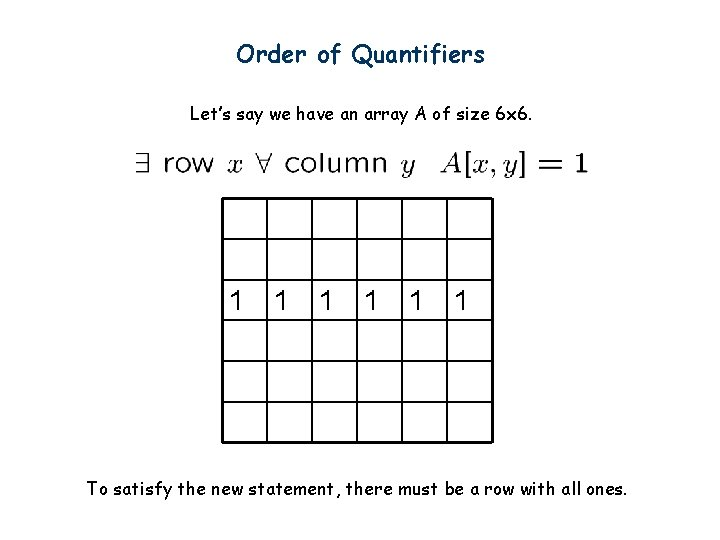Order of Quantifiers Let’s say we have an array A of size 6 x 6. 1 1 1 To satisfy the new statement, there must be a row with all ones.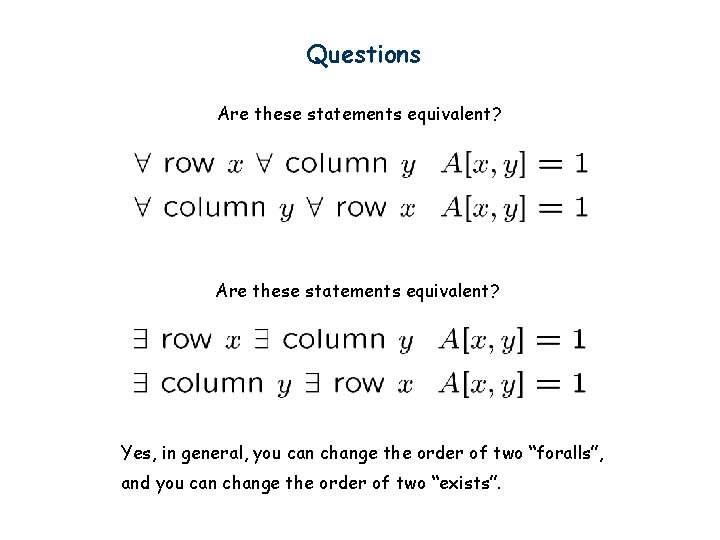Questions Are these statements equivalent? Yes, in general, you can change the order of two “foralls”, and you can change the order of two “exists”.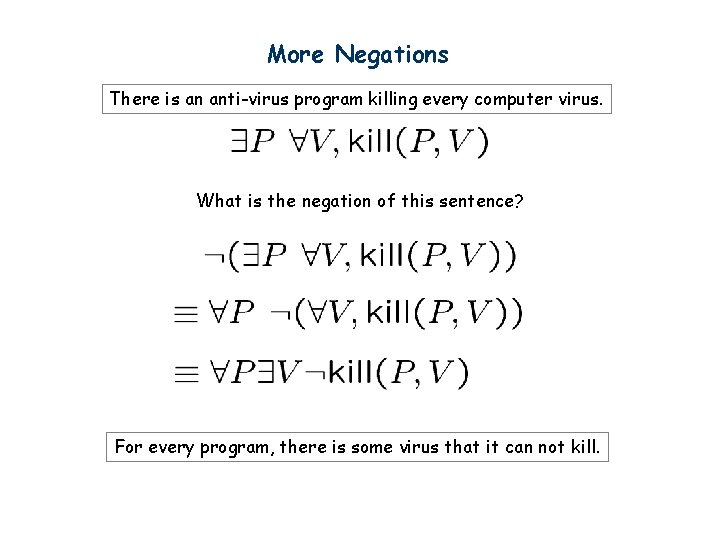More Negations There is an anti-virus program killing every computer virus. What is the negation of this sentence? For every program, there is some virus that it can not kill.Exercises 1. There is a smallest positive integer. 2. There is no smallest positive real number. 3. There are infinitely many prime numbers.Exercises 1. There is a smallest positive integer. 2. There is no smallest positive real number. 3. There are infinitely many prime numbers.